## Social Question# What do these symbols in maths mean.

Asked by weeeee (62) April 13th, 2010

∑ and ∫

and not only that more importantly how do you use them, what sort of equations would you use them in so I can get an understanding of how they work.

Observing members: 0Composing members: 0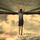The first one means the sum of. It is used a lot in statistics such as ∑x/n is the mean for a group of numbers which basically says “the mean is the sum of the numbers divided by the number of terms”.

The second is an integration sign. It is used to find the areas of curves. I won’t give an example of how this is done as its quite complicated to explain on first terms, but just look up integration and calculus on google/wikipedia.

Ame_Evil (3041)“Great Answer” (2) Flag as…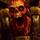sum and integral

ragingloli (44886)“Great Answer” (1) Flag as…@ragingloli
Go onto http://www.khanacademy.org/ and find questions that would involve them so I can do some, I about what they do and how to use them more than what they’re called.

Or you Ame.

weeeee (62)“Great Answer” (0) Flag as…@weeeee
These should be taught in your math class at school anyway, so why not ask your teacher, who can explain this to you better than some site on the internet?

ragingloli (44886)“Great Answer” (1) Flag as…@ragingloli
Not in school and can’t remember learning these.

weeeee (62)“Great Answer” (0) Flag as…@weeeee That site is very complicated. But check out the statistics section for ∑ (also called sigma) and the calculus section for the integral.

Ame_Evil (3041)“Great Answer” (0) Flag as…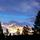∑ is sum. Underneath the symbol should be n= a number (It could be any letter, but n is used most often.), and another number (or infinity) should be abover the symbol. The n=some number is the starting point, and the top number is the ending point of the sum. To the right of it should be a function that includes n. So, replace n with the starting point, and evaluate the function. Then, replace n with the next number, evaluate the function, and add it to your previous result. Keep doing this until you have gone all the way through the last number.

For example, ∑4n where n goes from 3 to 6 is (4)(3)+(4)(4)+(4)(5)+(4)(6)=12+16+20+24=72.

I watched the first part of both of these videos, and they look like they provide a good explanation of sigma notation (the ∑ symbol is the Greek letter sigma). The sound quality is poor in the videos, but if you can get past that, they should help you understand how to do summations.

Your other symbol, ∫ is the integral. To be able to do integration, you really need to study calculus. Once again, I only looked at the first couple minutes of it, but this video looks like it will give you a decent explanation of the integral. It is enough to give you an idea of what is going on, but without studying the calculus that precedes integration, you really won’t be able to get a firm grasp of it.

bobbinhood (5894)“Great Answer” (2) Flag as…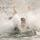You can take an entire semester of college calculus that’s only about the meaning of the integral sign. If you really want to understand the intricacy and power of both the summation (sigma) sign and the integral sign, you’d need to take a class. It’s not something that can be adequately summarized in an answer on Fluther or by watching an internet video.

hannahsugs (3238)“Great Answer” (1) Flag as…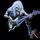Sigma is generally used in an equation to tell you the value it’s symbolizing is the sum of something. So for example, sigma x means adding up all of the values of x (which would likely be given in a table form somehow, and these are measured values for a statistical equation). It would be most commonly seen in standard deviation and variance equations. Findin the standard deviation of a data set would involve adding all the values, and adding some multiplied values, which is what sigma symbolizes

Hexr (475)“Great Answer” (0) Flag as…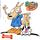In my math education (somewhat extensive), the integral has proven to be one of the kore powerful mathematical tools and certainly can’t been summed up by someone giving you a couple arbitrary problems from the Internet. If you’re truely interested, take a course.

PrancingUrchin (1939)“Great Answer” (0) Flag as…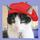Start out by checking on tangents to arcs and what happens when they approach each other.

gailcalled (54536)“Great Answer” (0) Flag as…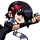I’m assuming that you are just entering the integral section of your first Calculus course. In addition to what has already been said, the integral and summation signs are related. An integral is a limit of a sum. More specifically, an integral is the limit as n goes to infinity of the sum, from 1 to n. It makes more sense when written out in notation…

Ivan (13439)“Great Answer” (0) Flag as…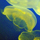Bah. Stop overcomplicating things @prancingurchin, @hannahsugs. No need to spook off someone who clearly wants to learn, math doesn’t need to be made out as hard just so you can be know it all snobs who won’t share knowledge. Thats just poor form, if you dont want to answer the question then don’t.

The ∫ sign is for integral.. it simply denotes the area under a curve, like the brown area here.

It would be used for example to find the area under the curve for a line equation like y=x^2 (like in the above link).

The ∑ sign is large sigma, which usually denotes a sum. A sum is simply a short way of writing something like 1+1+1+1+1+1+1…..

1+1+1+1… would be written as ∑ 1

you can complicate it by letting the thing being summed be variable, and set limits on how much you want to sum, for example
1+2+3+4 = ∑ n (from n=1 to 4)

It’s much like a programming function where you put in a number and let it run, but you can also use it as short hand notation as not to have to write out long sums. Another upside with the ∑ notation is that you can write infinetly long sums, something you can’t really do by writing out all the terms.

nisse (1986)“Great Answer” (1) Flag as…Here are some really basic (and good) video tutorials on integration and sums which answer your questions. You can find a lot more great math video tutorials on more advanced integration and sums on youtube.

nisse (1986)“Great Answer” (0) Flag as…